### Introduction to Earth Data Analysis

1. Get Started
1. Learn Git/Github for team collaboration
2. Install Python via Anaconda
3. Install Python IDE (Jupyter Notebook, Visual Studio Code)
4. Python variables and data types
5. Install and use Python packages
6. Get familiar with Numpy for data manipulations
7. Get familiar with Matplotlib for plotting arrays
8. Get familiar with Pandas to analyze tabular data
2. Get familiar with text file formats
1. Use basic Markdown syntax to format text in Jupyter Notebook files
2. Get familiar with text file formats - CSV, .txt, YAML
3. Read/Write data from text files using Python
4. Read/Write data from text files using Pandas
5. Deal with missing data in Pandas
3. Spatial Data Analysis
1. Read multi-layered raster data (.tif / .hdf / .nc) in Python
2. Read vector data (shapefiles) using geopandas
3. GIS in Python
4. Time-series Analysis using Pandas
2. Work with `Datetime` formats
3. Resample time series
4. Filtering/smoothing time-series with Pandas
5. Plot time series
5. How to plot topographic high-resolution geospatial maps

## Recent postsHow can you link unsplash images directly to your blog without hosting it locally.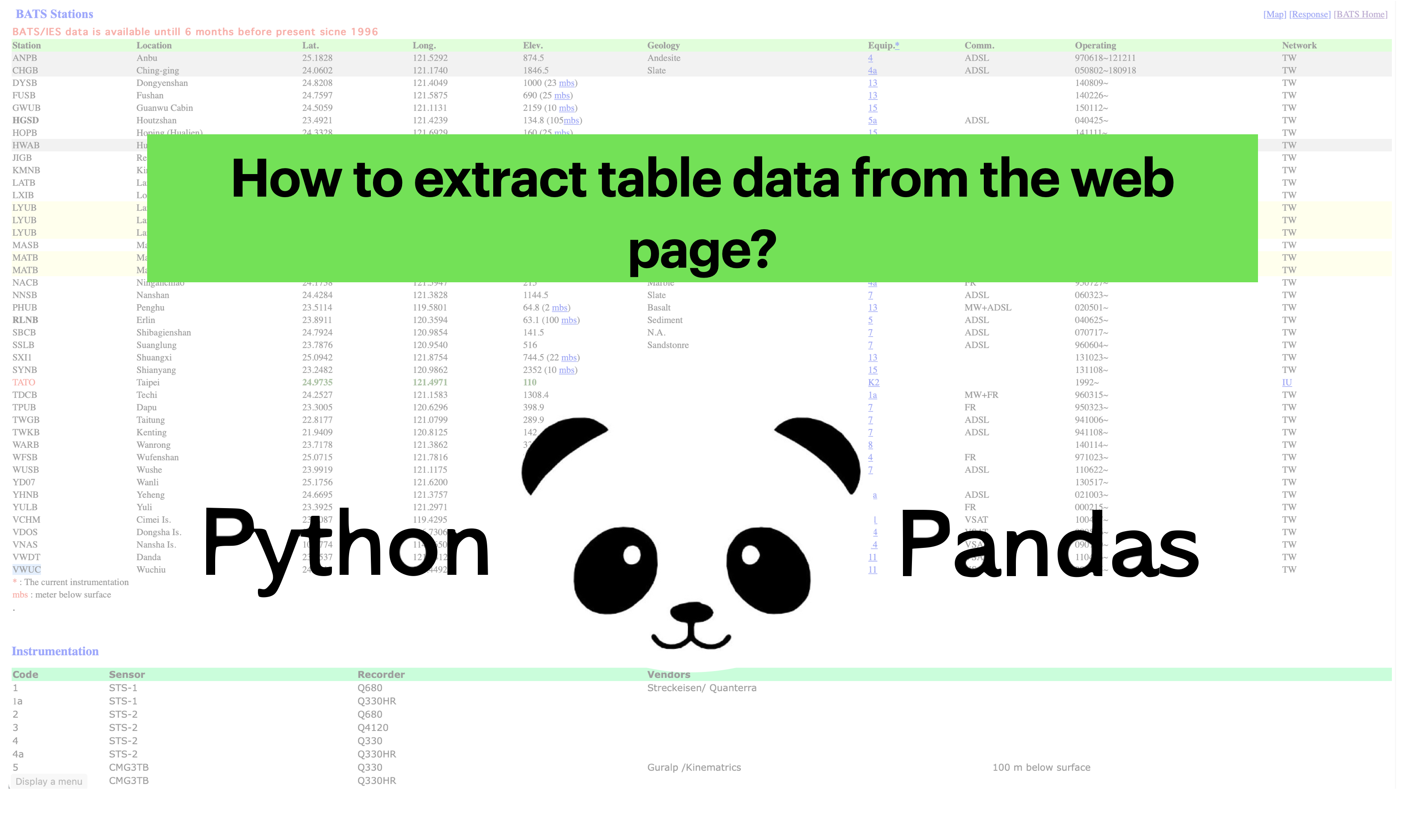## How to extract station data from the web page

Uses pandas to read the html page and extract the html data into pandas dataframe## How to plot the earthquakes data on a topographic map

Read the earthquake data from a csv file and overlay on a topographic map using PyGMT.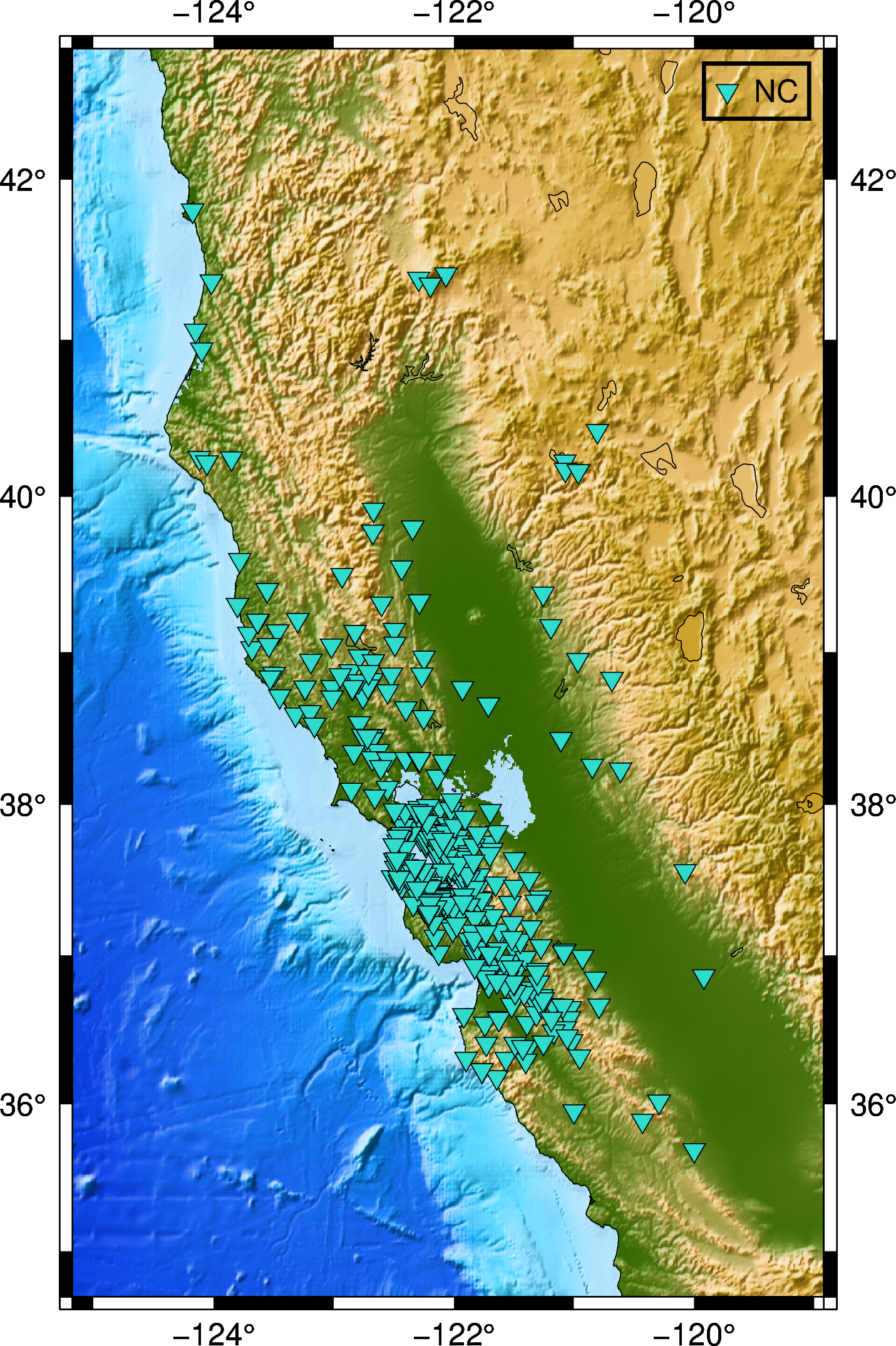## How to automatically enquire the availability of seismic data using obspy

In this post, we will see how can we retrive the available seismic waveforms information for a given network, station, channel and client in a given period o...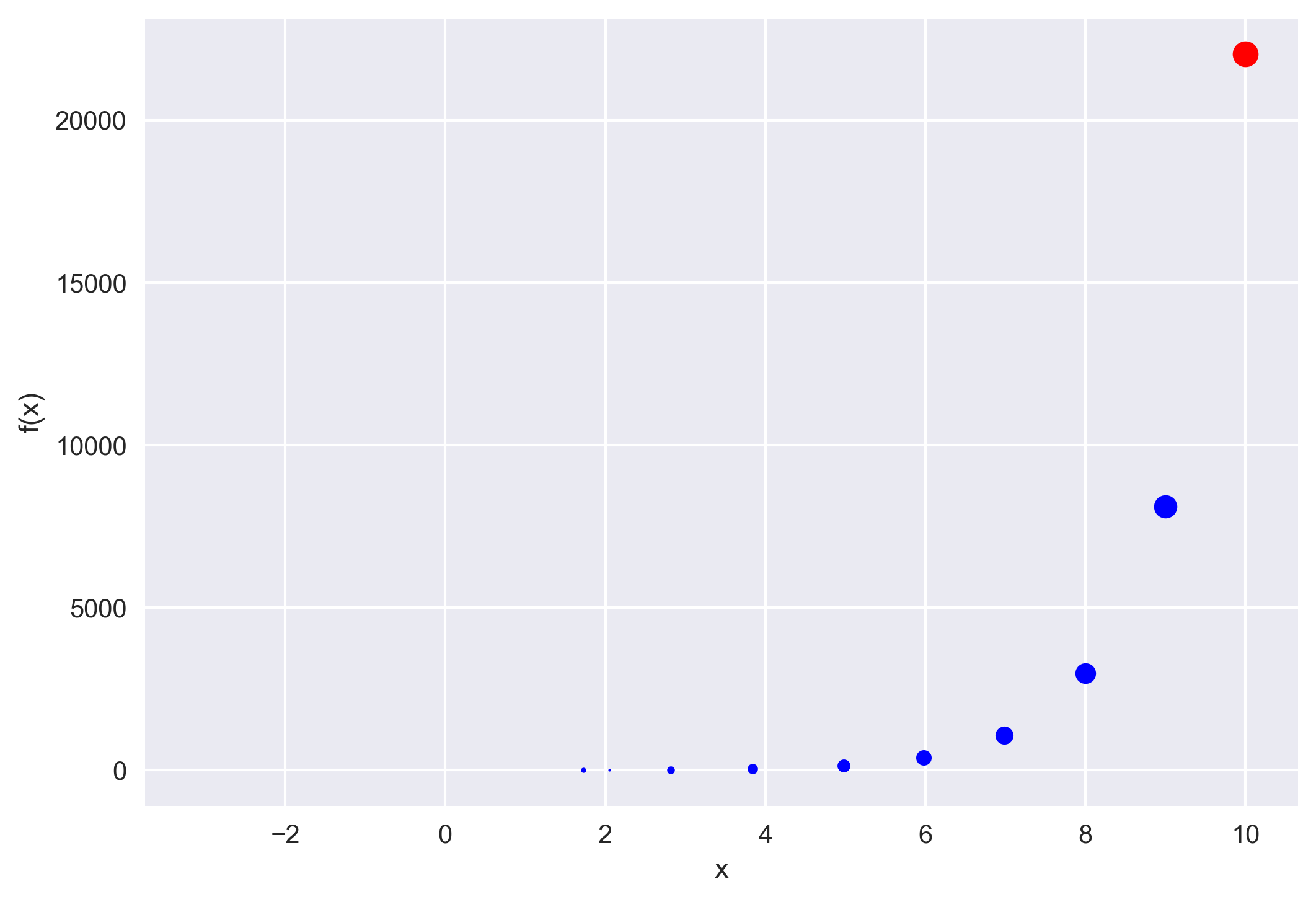## How to implement the iterative newton–raphson method to find roots of a function in python

The Newton–Raphson method (commonly known as Newton’s method) is developed for finding roots of a given function or polynomial iteratively. We show two examp...## How to host a http server on your linux computer to share files

Is the signal denoising using a wavelet effective? We will implement the wavelet-based signal denoising using the MATLAB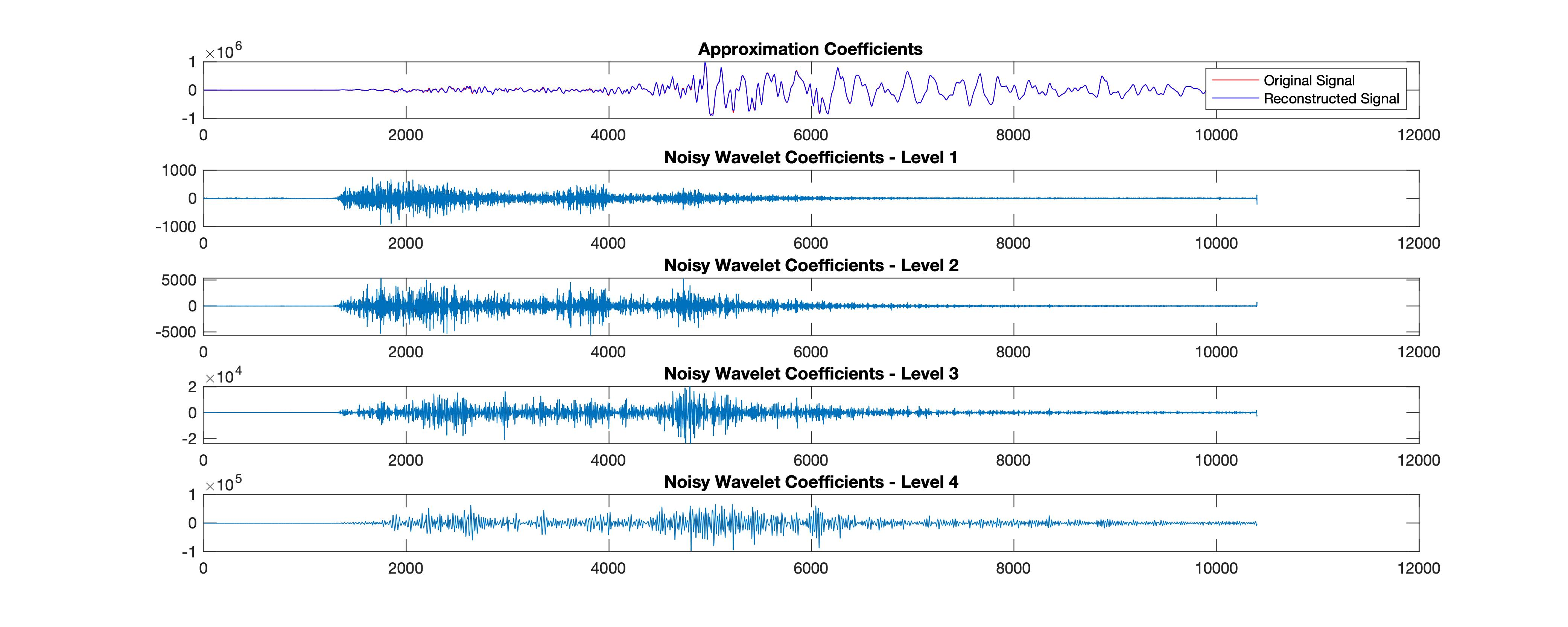## How effective is the signal denoising using the matlab based wavelet analysis

We see how to download seismic waveforms, convert them into mat format from mini-seed and then perform denoising using wavelet analysis. We first performed w...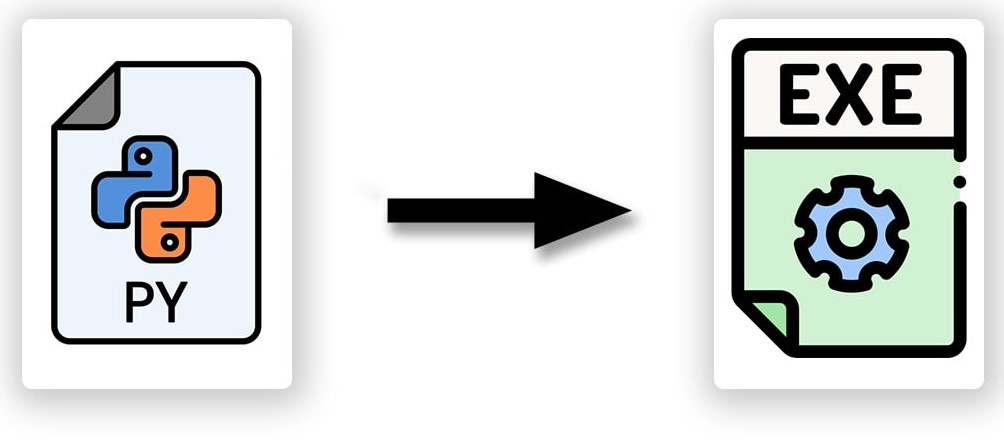## Make your python script executable from anywhere in linux

Follow the instructions to make your python script executable from anywhere in Linux system.## How to securely send data from local client to remote server using paramiko (codes included)

Paramiko module can be used in Python to securely send data from the local client to the remote server. It is analogous to the SSH and SCP in Linux.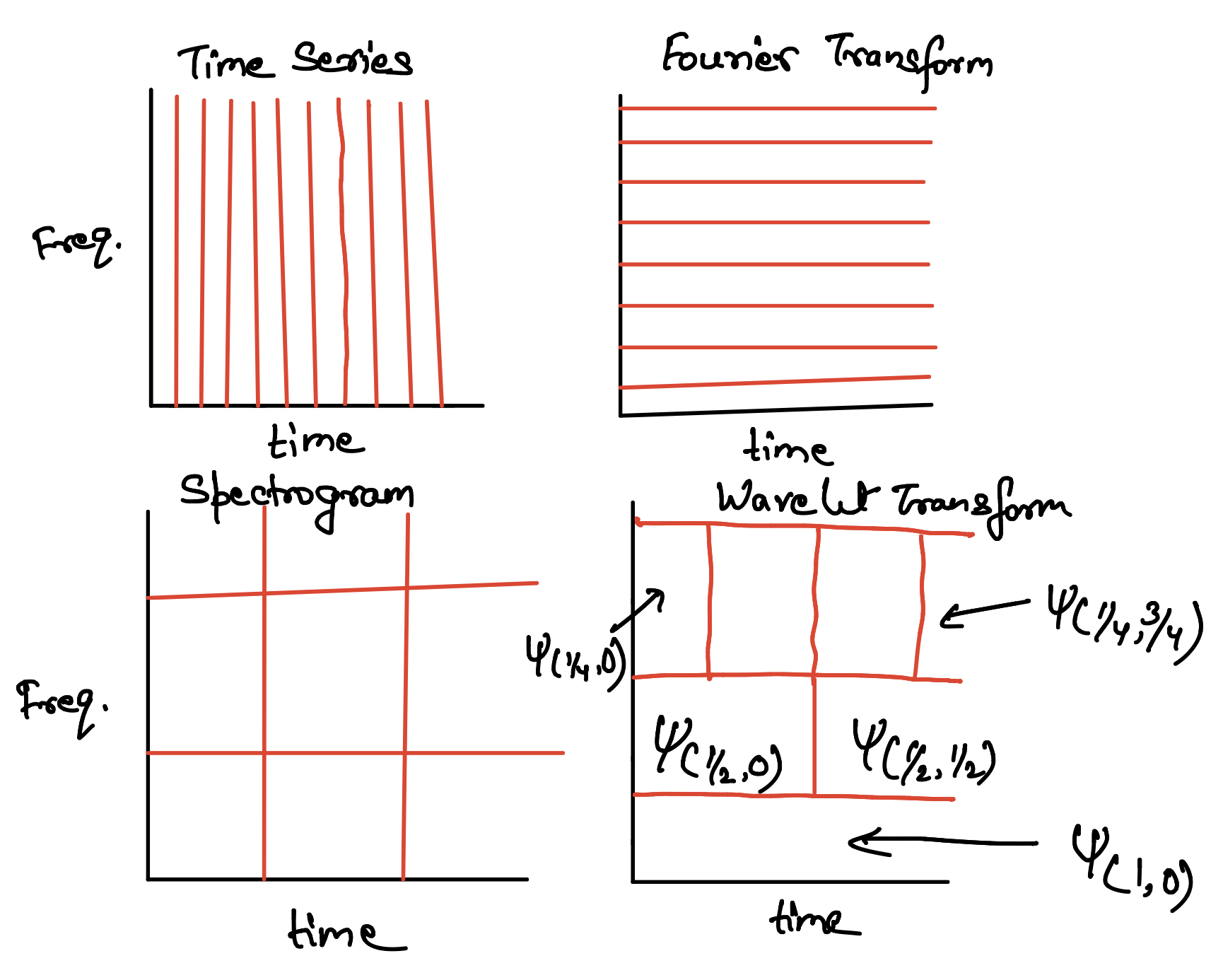## Towards multi-resolution analysis with wavelet transform

We will learn the basic concepts of wavelet tranform and multi-resolution analysis starting from the Fourier Transform, and Gabor Transform.## Empirical orthogonal function analysis to inspect the spatial coherency in the geospatial data (codes included)

Empirical Orthogonal Functions analysis decomposes the continuous space-time field into a set of orthogonal spatial patterns along with a set of associated u...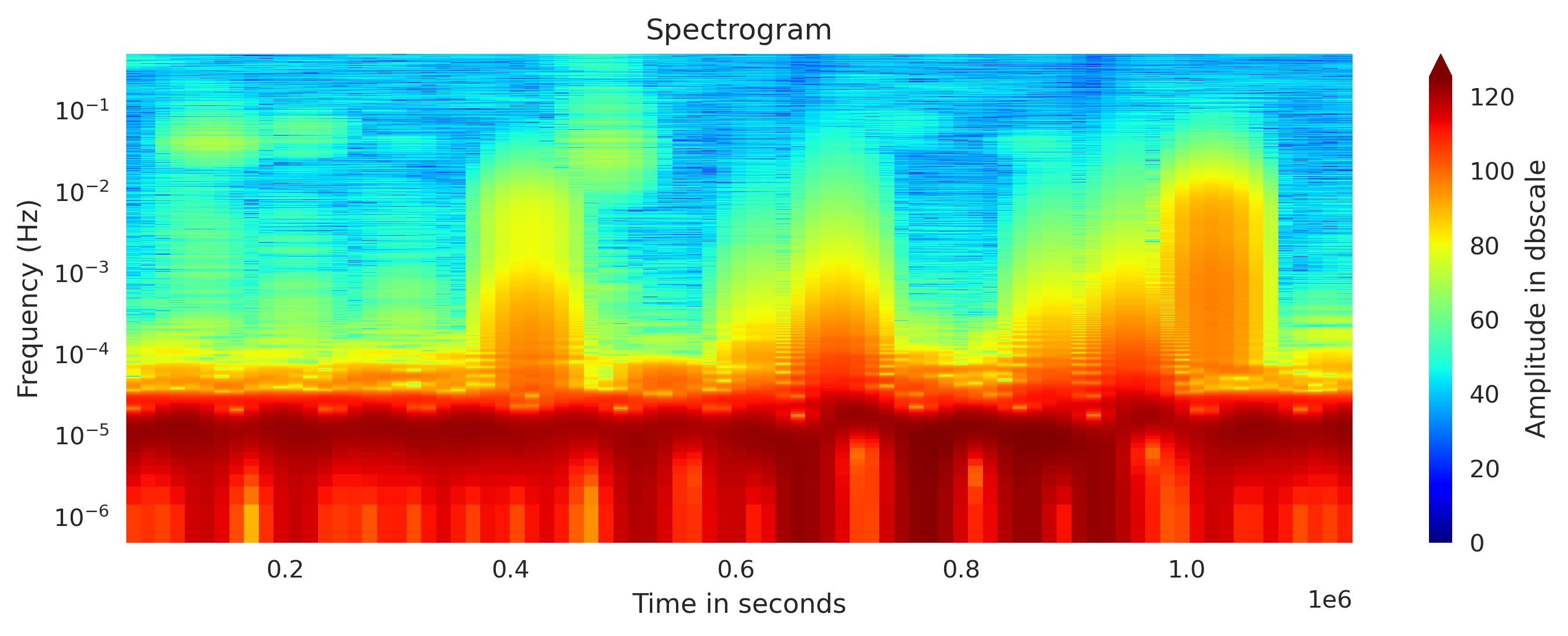## Concatenating daily seismic traces into one miniseed file (codes included)

I concatenate the daily seismic traces for 15 days into one miniseed file for further analysis. Then I obtained the spectrogram of the 15 days seismic data. ...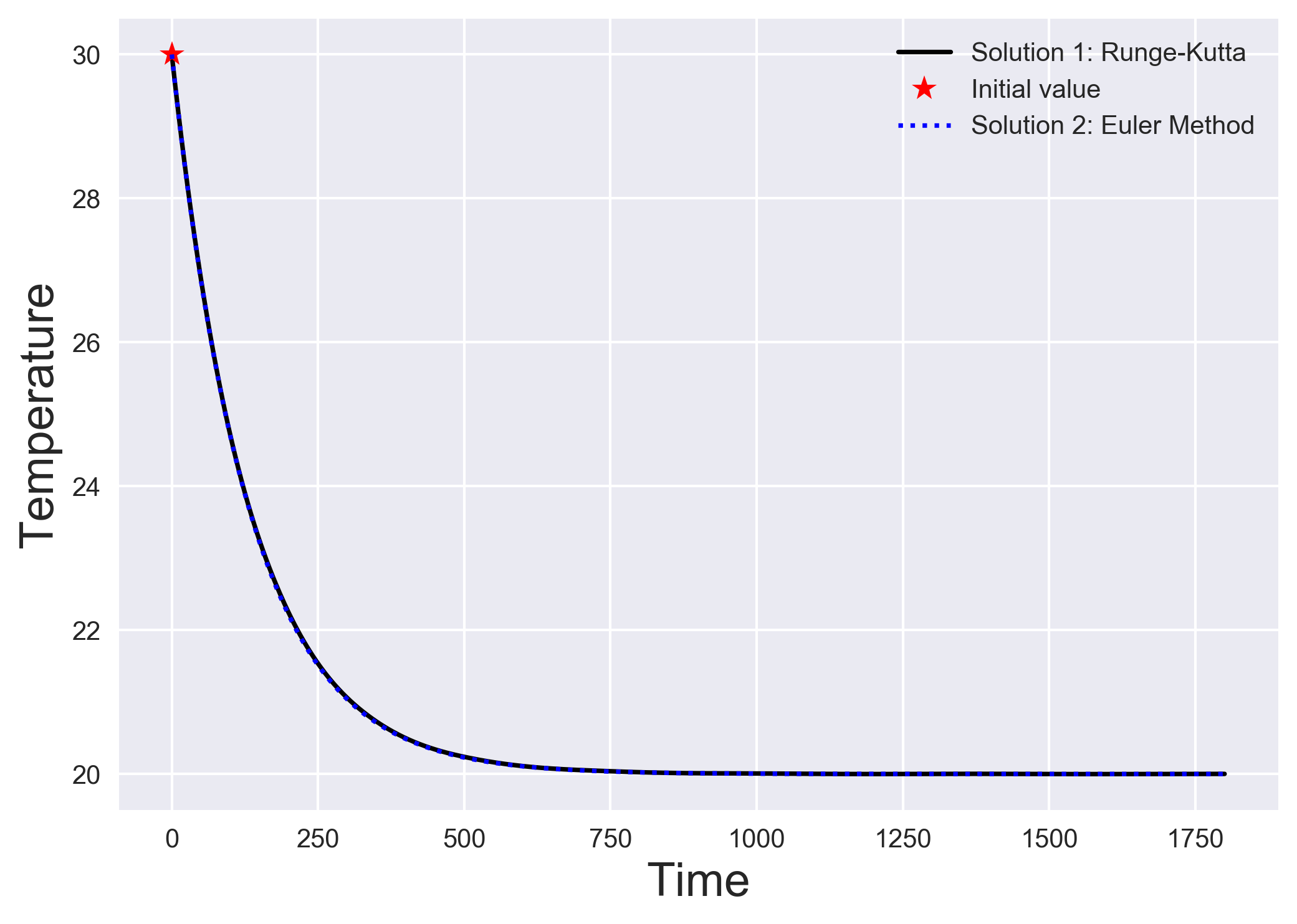## Numerically solving initial value problems using the euler method

The simplest algorithm to solve a system of differential equations is the Euler method. We understand the Euler method by looking into a simple heat transfer...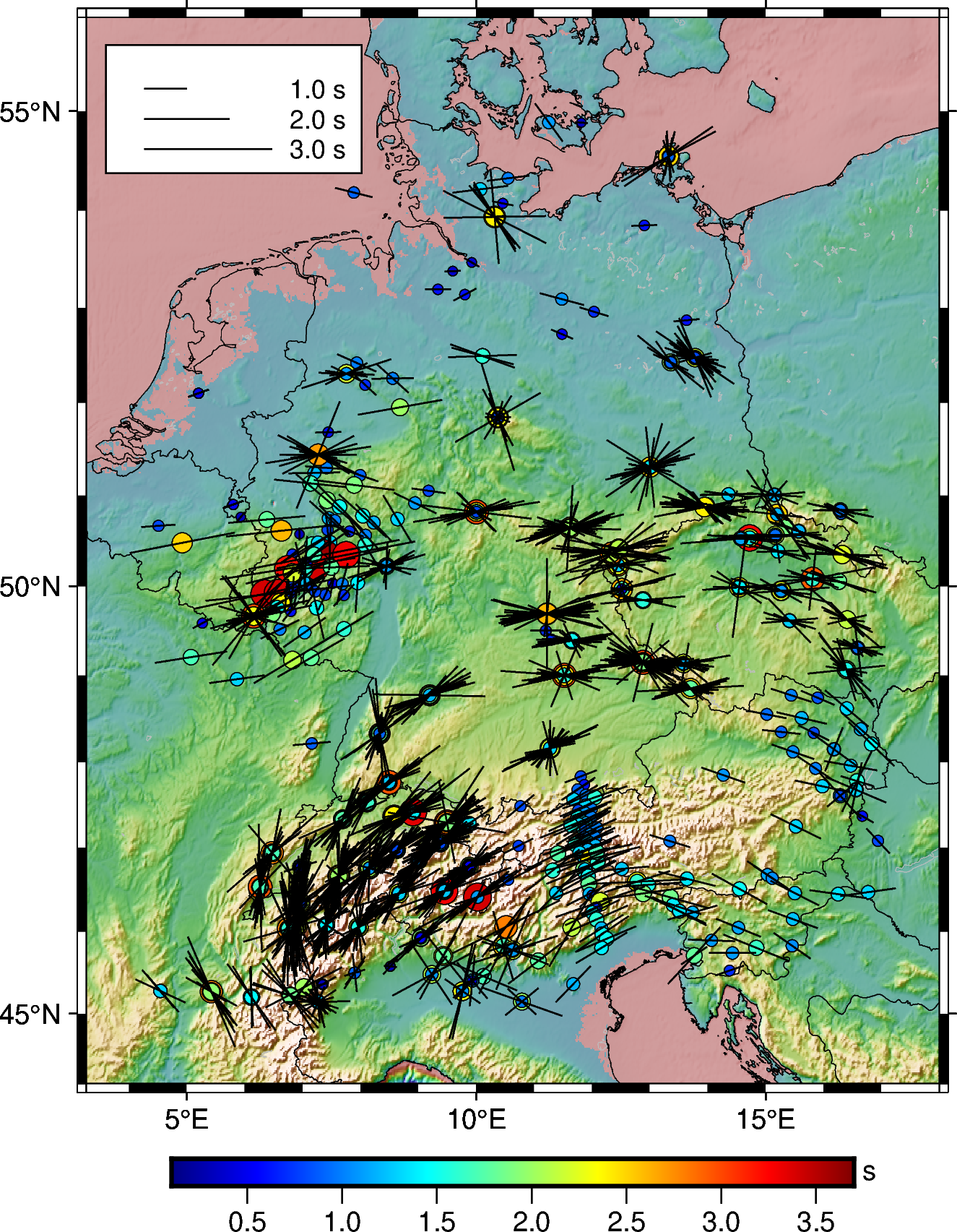## How to plot shear-wave splitting measurements using pygmt

How you can plot the shear-wave splitting measurements from splitting database using PyGMT.How can you link unsplash images directly to your blog without hosting it locally.## How to extract station data from the web page

Uses pandas to read the html page and extract the html data into pandas dataframe## How to plot the earthquakes data on a topographic map

Read the earthquake data from a csv file and overlay on a topographic map using PyGMT.## How to automatically enquire the availability of seismic data using obspy

In this post, we will see how can we retrive the available seismic waveforms information for a given network, station, channel and client in a given period o...## How to implement the iterative newton–raphson method to find roots of a function in python

The Newton–Raphson method (commonly known as Newton’s method) is developed for finding roots of a given function or polynomial iteratively. We show two examp...## How to host a http server on your linux computer to share files

Is the signal denoising using a wavelet effective? We will implement the wavelet-based signal denoising using the MATLAB## How effective is the signal denoising using the matlab based wavelet analysis

We see how to download seismic waveforms, convert them into mat format from mini-seed and then perform denoising using wavelet analysis. We first performed w...## Make your python script executable from anywhere in linux

Follow the instructions to make your python script executable from anywhere in Linux system.## How to securely send data from local client to remote server using paramiko (codes included)

Paramiko module can be used in Python to securely send data from the local client to the remote server. It is analogous to the SSH and SCP in Linux.## Towards multi-resolution analysis with wavelet transform

We will learn the basic concepts of wavelet tranform and multi-resolution analysis starting from the Fourier Transform, and Gabor Transform.## Empirical orthogonal function analysis to inspect the spatial coherency in the geospatial data (codes included)